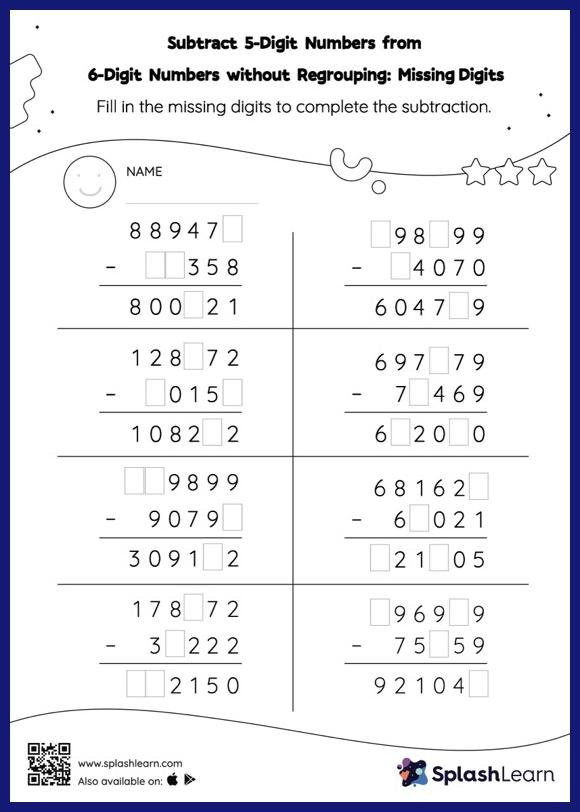# Subtract 5-Digit Numbers from 6-Digit Numbers without Regrouping: Missing Digits Worksheet

Home > Subtract 5-Digit Numbers from 6-Digit Numbers without Regrouping: Missing DigitsIn this worksheet, students must find the missing number using the count back method or the relationship between addition and subtraction. To solve subtract 5-digit numbers from 6-digit numbers without regrouping worksheet, they do not need to regroup the numbers. In each problem, the numbers are laid out in the vertical format. In this format, pair of digits in each successive place are tackled one by one. This helps students follow a structured approach.